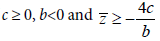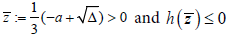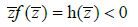## Research and Reports on Mathematics

All submissions of the EM system will be redirected to Online Manuscript Submission System. Authors are requested to submit articles directly to Online Manuscript Submission System of respective journal.

Research Article, Res Rep Math Vol: 2 Issue: 4

# Sufficient condition of the Hopf bifurcation

Amine Bernoussi*

Department of Mathematics, Faculty of Science, Ibn Tofail University, Kenitra, Morocco

*Corresponding Author : Amine Bernoussi
Department of Mathematics, Faculty of Science, Ibn Tofail University, BP 133, 14000, Kenitra, Morocco
E-mail: [email protected]

Received: September 15, 2018 Accepted: December 04, 2018 Published: December 11, 2018

Citation: Bernoussi A (2018) Sufficient Condition of the Hopf Bifurcation. Res Rep Math 2:4

## Abstract

In this paper we propose a new sufficient condition for the Hopf bifurcation of equilibrium is derived for nonlinear autonomous differential equations with delay in three dimension, by analysis the characteristic equation.

## Introduction

In this paper, we propose the following sufficient condition for the local Hopf bifurcation for a differential equation system with delay the form:The initial condition for the above system iswith φ=(φ1, φ2, φ3) where φi∈C(i=1,2,3), such that φi(θ) ≥ 0(-τ≤θ≤ 0,i=1,2,3). Here C denotes the Banach space C([-τ,0]R) of continuous functions mapping the interval [-τ,0] into R, equipped with the supremum norm. The non-negative cone of C is defined as C+= C([-τ,0], R+), where R+={x∈ R|x ≥ 0}. We here assume that f: R3→ R is a locally Lipschitz continuous function on R3 Suppose that the equation (1) has a equilibrium point P*; and the characteristic equation of the equation (1) around the equilibrium point P* takes the general form:Withand## The main results are as follows

Theorem 1 (Amine Bernoussi) Assume that the equilibrium P* of system (1) is locally asymptotically stable for τ=0:

IfThen there exists a positive τ0 such that, when τ∈[0,τ0) the steady state P* is locally asymptotically stable, and a Hopf bifurcation occurs as τ passes through τ0; where τ0 is given byand ω0 is the least simple positive root of equation (5), and c, b and ͞z are defined in lemma 2 and equation (5). further we haveThe organization of this paper is as follows. In section 2, we offer basic results for the prove of theorem 1. In section 3, we establish the local asymptotic stability and the Hopf bifurcation of the equilibrium P* and prove theorem 1. Finally a discussion and conclusion is offered in section 4.

## Basic Results

We offer some basic results for the prove of theorem 1. Now we return to the study of equation (2) with τ>0. Equation (2) has a purely imaginary root iω, with ω>0:

Δ(iω, τ)=0

if and only ifSquaring and adding the squares together, we obtainwithwhere A, B, C, D, E and F are given by (2).

Letting z=ω2, equation (5) becomes the following cubic equationLemma 2  Define(i) If c < 0, then equation (6) has at least one positive root.

(ii) If c ≥ 0 and Δ ≤ 0, then equation (6) has no positive roots.

(iii) If c ≥ 0 and Δ>0, then equation (6) has positive roots if and

only ifFrom lemma 2, we have the following lemma.

Lemma 3 Assume that the equilibrium P* of system (1) is locally asymptotically stable for τ=0

(i) If one of the following:

(N1) c ≥ 0, and Δ ≤ 0,

(N2) c ≥ 0, Δ>0, and ͞z ≤ 0,

(N3) c ≥ 0, Δ>0, and h( ͞z )>0,

is true, then all roots of equation (2) have negative real parts for all τ ≥ 0.

(ii) If c<0, or c ≥ 0, Δ>0, ͞z >0, and h( ͞z )≤ 0, then all roots of equation (2) have negative real parts whenwhere Δ and ͞z >0 are defined in lemma 2

## Sufficient Condition of the Hopf Bifurcation

In this section, we establish the condition sufisent of the Hopf bifurcation of the equilibrium P*.

Theorem 4 Assume that the equilibrium P* of system (1) is locally asymptotically stable for τ=0.

IfThen there exists a positive τ0 such that, when τ∈[0, τ0) the steady state P* is locally asymptotically stable, and a Hopf bifurcation occurs as τ passes through τ0, where τ0 is given byand ω0 is the least simple positive root of equation (5), and c, b and z are defined in lemma 2 and equation (5). further we haveProof. Consider the equationthe discriminant Δ1 of the equation (8) is given byor by the hypothes c ≥ 0 andtherefore equation (8) admits two solutionsthen we havethe hypothesesimplies thatThereforeOn the other since from the hypotheses b<0 then Δ>0 then the hypotheses (iii) of the Lemma 2 is satisfied. Hence the equation (6) has at least one positive solution, more Lemma 3 implies that all roots of equation (2) have negative real parts when τ [0, τ0).

Now we will determine τ0.

Without loss of generality, we assume that the equation (6) has three positive roots, denoted by z1, z2 and z3, respectively.

Then equation (5) has three positive roots, sayAccording to equations (3) and (4) we haveThen ±iωl is a pair of purely imaginary roots of equation (2) withclearly,Thus, we can defineNext we need to guarantee the transversality condition of the Hopf bifurcation theorem [1-4].AndLet u(τ) and ω(τ) satisfying u(τ0)=0, and ω(τ0)=ω0. By differentiating the equations (9) and (10) with respect to τ and then set τ=τ0. Doing this, we getWhereSolving forwe getTherefore we haveNote that ifh(0)=c≥0.

Thus ifwe have the transversality condition:Ifand close to τ0, then equation (2) has a root λ(τ) = u(τ)+iω(τ) satisfying u(τ)>0, which contradicts (ii) of Lemma 3. This completes the proof.

Remark 5 Our main result also valid for any differential equation of higher dimension three provided that the study of the characteristic equation of the equation reduces to study the equation (2) and more generally all the problems in Rn has equation of the form [5-7]:## Conclusion

In this work, we presented a mathematical analysis for a nonlinear autonomous differential equation with delays in three dimensions. The originality of this work is to have a new sufficient condition for the Hopf bifurcation of equilibria. On the other hand, the main results can be generalized to differential equation of dimension greater than three (see Remark 5).

## References## Explore SciTechnol

###### Citations : 5

Research and Reports on Mathematics received 5 citations as per Google Scholar report

## Recommended Conferences

### 7th Annual Meet on Advances in Physics, Mathematics and Applied Science

Budapest, Hungary